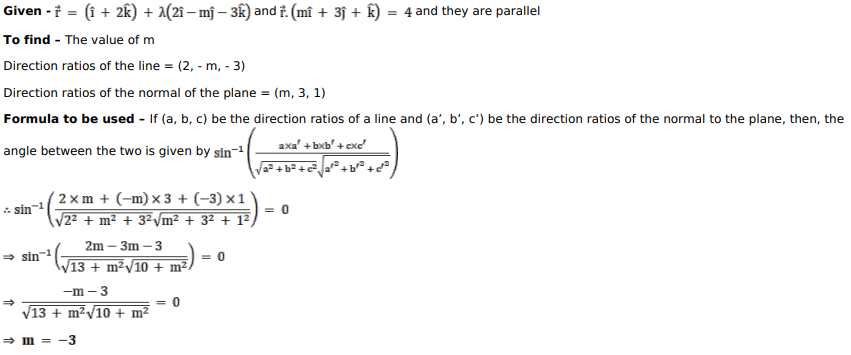# Solve this following

Question:

Find the value of $m$ for which the line $\vec{r}=(\hat{i}+2 \hat{k})+\lambda(2 \hat{i}-m \hat{j}-3 \hat{k})$ is parallel to the plane $\vec{r} \cdot(m \hat{i}+3 \hat{j}+\hat{k})=4$.

Solution: